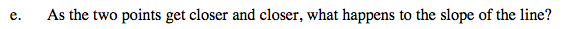### Home > PC > Chapter 8 > Lesson 8.2.4 > Problem8-126

8-126.
1. Given the function f(x) = 10 − x2, find the slope of the line between the following pairs of points. Homework Help ✎

1. (2, f(2)) and (4, f(4))

2. (2, f(2)) and (3, f(3))

3. (2, f(2)) and (2.5, f(2.5))

4. (2, f(2)) and (2.1, f(2.1))

5. As the two points get closer and closer, what happens to the slope of the line?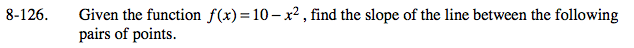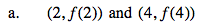$m=\frac{f(4)-f(2)}{4-2}$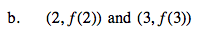$m=\frac{f(3)-f(2)}{3-2}$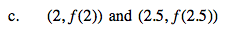$m=\frac{f(2.5)-f(2)}{2.5-2}$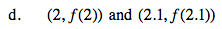$m=\frac{f(2.1)-f(2)}{2.1-2}$